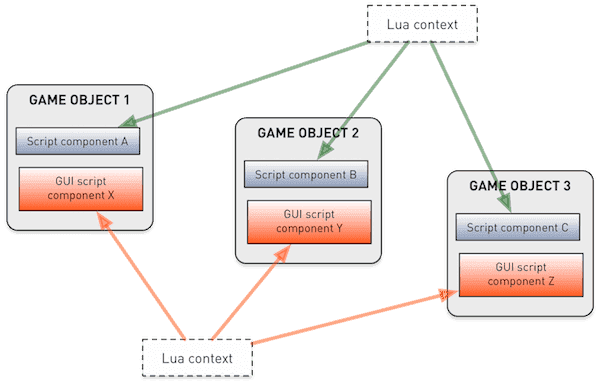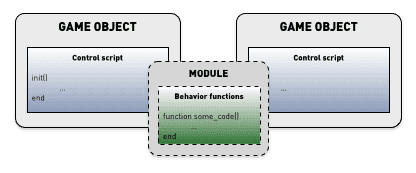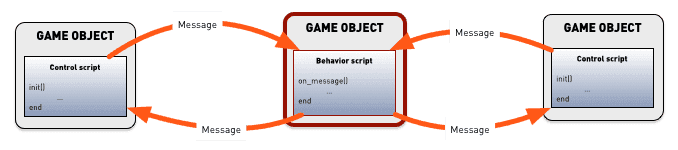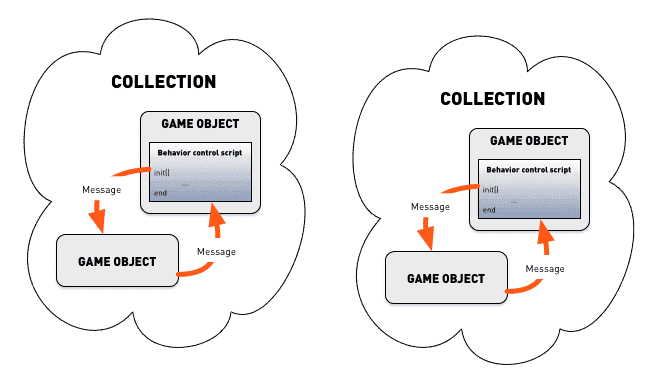# Defold 中的 Lua

Defold 引擎嵌入了 Lua 语言用以编写脚本. Lua 是一种轻量级脚本语言, 不但功能强大, 而且速度块, 易于嵌入. 它被广泛运用于游戏脚本编程. Lua 程序是简单过程语法. 脚本动态地输入并且运行于字节码解释器上. 它包含了自动内存管理和垃圾处理功能.

## Lua 版本

Defold 包含所有 Lua 5.1 标准库 连同 socket 和少量操作系统功能库:

• base (`assert()`, `error()`, `print()`, `ipairs()`, `require()` 等等)
• coroutine
• package
• string
• table
• math
• io
• os
• debug
• socket (参考 LuaSocket)
• bitop (参考 BitOp)

## 语法

``````--[[

--]]

a = 10
b = 20 ; c = 30 -- 一行定义俩变量

if my_variable == 3 then
call_some_function(true) -- 这是一个行注释
else
call_another_function(false)
end
``````

## 变量和数据类型

Lua 是动态类型语言也就是说变量没有固定类型, 但是值有类型. 不像固定类型语言, 可以给变量规定一个类型. Lua 里有8种值类型:

`nil`

``````print(my_var) -- 输出 'nil' 因为 'my_var' 还没赋值
``````
boolean

``````flag = true
if flag then
print("flag is true")
else
print("flag is false")
end

if my_var then
print("my_var is not nil nor false!")
end

if not my_var then
print("my_var is either nil or false!")
end
``````
number

``````print(10) --> 输出 '10'
print(10.0) --> '10'
print(10.000000000001) --> '10.000000000001'

a = 5 -- 整数
b = 7/3 -- 浮点数
print(a - b) --> '2.6666666666667'
``````
string

`\a` 响铃
`\b` 退格
`\f` 表格填充
`\n` 下一行
`\r` 回车
`\t` 水平制表符
`\v` 垂直制表符
`\\` 反斜杠
`\"` 双引号
`\'` 单引号
`\[` 左中括号
`\]` 右中括号
`\ddd` 数字占位符, 其中 ddd 表示3个 浮点 数字
``````my_string = "hello"
another_string = 'world'
print(my_string .. another_string) --> "helloworld"

print("10.2" + 1) --> 11.2
print(my_string + 1) -- error, can't convert "hello"
print(my_string .. 1) --> "hello1"

print("one\nstring") --> one
--> string

print("\097bc") --> "abc"

multi_line_string = [[
Here is a chunk of text that runs over several lines. This is all
put into the string and is sometimes very handy.
]]
``````
function

``````-- 赋值 'my_plus' 为函数
my_plus = function(p, q)
return p + q
end

print(my_plus(4, 5)) --> 9

-- 函数 'my_mult' 标准声明
function my_mult(p, q)
return p * q
end

print(my_mult(4, 5)) --> 20

-- 把函数 'func' 用作参数
function operate(func, p, q)
return func(p, q) -- Calls the provided function with parameters 'p' and 'q'
end

print(operate(my_plus, 4, 5)) --> 9
print(operate(my_mult, 4, 5)) --> 20

return function(a)
return a + n
end
end

``````
table

``````-- 初始化表中序列
weekdays = {"Sunday", "Monday", "Tuesday", "Wednesday",
"Thursday", "Friday", "Saturday"}
print(weekdays) --> "Sunday"
print(weekdays) --> "Thursday"

-- 初始化表作为记录使用
moons = { Earth = { "Moon" },
Uranus = { "Puck", "Miranda", "Ariel", "Umbriel", "Titania", "Oberon" } }
print(moons.Uranus) --> "Ariel"

-- 使用构造符号 {} 创建一个表
a = 1
t = {}
t = "first"
t[a + 1] = "second"
t.x = 1 -- same as t["x"] = 1

-- 迭代表中键值对
for key, value in pairs(t) do
print(key, value)
end
--> 1   first
--> 2   second
--> x   1

u = t -- u 和 t 都保存了对表的引用
u = "changed"

for key, value in pairs(t) do --再次迭代t!
print(key, value)
end
--> 1   changed
--> 2   second
--> x   1
``````
userdata

## Operators

``````a = -1
print(a * 2 + 3 / 4^5) --> -1.9970703125
``````

Lua 能在运行时按需自动转换数字与字符串. 对字符串的数学操作都会进行数字化尝试:

``````print("10" + 1) --> 11
``````

`<` (小于), `>` (大于), `<=` (小于等于), `>=` (大于等于), `==` (相等), `~=` (不等). 这些操作都返回 `true` 或者 `false`. 不同类型值使用不同比较方法. 如果类型相同, 就比较它们的值的大小. Lua 使用引用对比表, 用户数据, 和函数. 引用同一对象的两个值是相等的.
``````a = 5
b = 6

if a <= b then
print("a is less than or equal to b")
end

print("A" < "a") --> true
print("aa" < "ab") --> true
print(10 == "10") --> false
print(tostring(10) == "10") --> true
``````

`and`, `or`, 和 `not`. `and` 遇见第一个参数是 `false` 的话就返回这个参数, 否则返回第二个参数. `or` 遇见第一个参数不是 `false` 就返回第一个参数, 否则返回第二个参数.
``````print(true or false) --> true
print(true and false) --> false
print(not false) --> true

if a == 5 and b == 6 then
print("a is 5 and b is 6")
end
``````

``````print("donkey" .. "kong") --> "donkeykong"
print(1 .. 2) --> "12"
``````

``````s = "donkey"
print(#s) --> 6

t = { "a", "b", "c", "d" }
print(#t) --> 4

u = { a = 1, b = 2, c = 3 }
print(#u) --> 0

v = { "a", "b", nil }
print(#v) --> 2
``````

## 流程控制

Lua 提供了常用的流程控制集.

if—then—else

``````a = 5
b = 4

if a < b then
print("a is smaller than b")
end

if a == '1' then
print("a is 1")
elseif a == '2' then
print("a is 2")
elseif a == '3' then
print("a is 3")
else
print("I have no idea what a is...")
end
``````
while

``````weekdays = {"Sunday", "Monday", "Tuesday", "Wednesday",
"Thursday", "Friday", "Saturday"}

-- 输出每一天
i = 1
while weekdays[i] do
print(weekdays[i])
i = i + 1
end
``````
repeat—until

``````weekdays = {"Sunday", "Monday", "Tuesday", "Wednesday",
"Thursday", "Friday", "Saturday"}

-- 输出每一天
i = 0
repeat
i = i + 1
print(weekdays[i])
until weekdays[i] == "Saturday"
``````
for
Lua 有两种 `for` 循环: 数值的和通用的. 数值的 `for` 需要 2 到 3 个参数而通用的 `for` 使用 迭代器 函数迭代表中所有内容.
``````-- 输出数字 1 到 10
for i = 1, 10 do
print(i)
end

-- 输出数字 1 到 10 而且每次步进 2 个值
for i = 1, 10, 2 do
print(i)
end

-- 输出数字 10 到 1
for i=10, 1, -1 do
print(i)
end

t = { "a", "b", "c", "d" }
-- 迭代序列输出内容
for i, v in ipairs(t) do
print(v)
end
``````
break 和 return

``````a = 1
while true do
a = a + 1
if a >= 100 then
break
end
end

return a + b
end

``````

## 本地, 全局和词法范围

``````function my_func(a, b)
-- 'a' 和 'b' 是函数本地变量并在函数范围内有效

do
local x = 1
end

print(x) --> nil. 'x' 在 do-end 范围之外无效
print(foo) --> nil. 'foo' 的定义在 'my_func' 之后
print(foo_global) --> "value 2"
end

local foo = "value 1"
foo_global = "value 2"

print(foo) --> "value 1". 'foo' 经过定义就变成文件范围本地有效了.
``````

``````local func2 -- 提前声明 'func2'

local function func1(a)
print("func1")
func2(a)
end

function func2(a) -- or func2 = function(a)
print("func2")
if a < 10 then
func1(a + 1)
end
end

function init(self)
func1(1)
end
``````

``````function create_counter(x)
-- 'x' is a local variable in 'create_counter'
return function()
x = x + 1
return x
end
end

count1 = create_counter(10)
count2 = create_counter(20)
print(count1()) --> 11
print(count2()) --> 21
print(count1()) --> 12
``````

## 变量覆盖

``````my_global = "global"
print(my_global) -->"global"

local v = "local"
print(v) --> "local"

local function test(v)
print(v)
end

function init(self)
v = "apple"
print(v) --> "apple"
test("banana") --> "banana"
end
``````

## Coroutines

``````-- 这就是协程
local function sequence(self)
coroutine.yield(120)
coroutine.yield(320)
coroutine.yield(510)
coroutine.yield(240)
return 440 -- 返回最后一个值
end

function init(self)
self.co = coroutine.create(sequence) -- 创建协程. 'self.co' 是线程对象
go.set_position(vmath.vector3(100, 0, 0)) -- 设置初始位置
end

function update(self, dt)
local status, y_pos = coroutine.resume(self.co, self) -- 继续运行协程.
if status then
-- 如果协程还没运行完, 则使用协程返回的位置值
go.set_position(vmath.vector3(100, y_pos, 0))
end
end
``````

## Defold 里的 Lua 上下文Defold 允许在多个游戏对象上使用相同的脚本组件. 相同脚本的本地变量在各个使用它的组件中共享.

``````-- 'my_global_value' 可以被所有脚本, gui 脚本, 渲染脚本以及模块 (Lua 文件) 访问
my_global_value = "global scope"

-- 此变量可以被所有使用本脚本文件的组件访问
local script_value = "script scope"

function init(self, dt)
-- 这个变量只在本组件中可以访问
self.foo = "self scope"

-- 这个变量只在init函数里而且必须在声明之后才可以访问
local local_foo = "local scope"
print(local_foo)
end

function update(self, dt)
print(self.foo)
print(my_global_value)
print(script_value)
print(local_foo) -- 在 init() 之外此变量不可访问
end
``````

## 性能考虑

``````local t = socket.gettime()
local table = {}
for i=1,2000 do
table[i] = vmath.vector3(i, i, i)
end
print((socket.gettime() - t) * 1000)

-- DEBUG:SCRIPT: 0.40388
``````

## 内存和垃圾收集

Lua 默认在后台自动运行垃圾收集功能以回收运行时分配出去的内存资源. 一次大量的垃圾回收会耗时很久所以最好的办法就是尽量减少垃圾积累:

• 本地变量会自动释放不会产生垃圾. (比如 `local v = 42`)
• 每一个 新的不重复的 字符串都是一个新对象. 代码 `local s = "some_string"` 就创建了新对象然后把引用赋给 `s`. 本地 `s` 不会产生垃圾, 但是字符串对象会. 同样字符串定义多次不会过多增加内存占用.
• 每次运行表的构造代码 (`{ ... }`) 就能创建新表.
• 执行 函数声明 就创建了闭包对象. (比如, 执行 `function () ... end`, 不是调用定义的还函数)
• 未知参数函数 (`function(v, ...) end`) 在函数每次被 调用 时为省略的参数提供存储表 (Lua 5.2 版之前, 或者不使用 LuaJIT).
• `dofile()``dostring()`
• Userdata 对象

``````-- 重置速度
self.velocity = vmath.vector3()
``````

``````print(collectgarbage("count") * 1024)       -- 88634
local v = vmath.vector3()
print(collectgarbage("count") * 1024)       -- 88704. 70 字节内存被分配
``````

``````print(collectgarbage("count") * 1024)       -- 88611
print(collectgarbage("count") * 1024)       -- 88633. 22 字节内存被分配
``````

``````-- 不使用 self.velocity = vmath.vector3() 带来的额外消耗
-- 而只把要使用的矢量属性清零即可
self.velocity.x = 0
self.velocity.y = 0
self.velocity.z = 0
``````

``````print(collectgarbage("count") * 1024)
``````

## 最佳实践Did you spot an error or do you have a suggestion? Please let us know on GitHub!